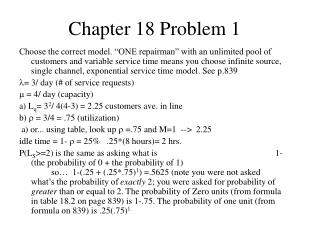DownloadDownload PresentationChapter 18 Problem 1

# Chapter 18 Problem 1

Download Presentation## Chapter 18 Problem 1

- - - - - - - - - - - - - - - - - - - - - - - - - - - E N D - - - - - - - - - - - - - - - - - - - - - - - - - - -
##### Presentation Transcript

1. Chapter 18 Problem 1 Choose the correct model. “ONE repairman” with an unlimited pool of customers and variable service time means you choose infinite source, single channel, exponential service time model. See p.839 = 3/ day (# of service requests)  = 4/ day (capacity) a) Lq= 32/ 4(4-3) = 2.25 customers ave. in line b)  = 3/4 = .75 (utilization) a) or... using table, look up  =.75 and M=1 --> 2.25 idle time = 1-  = 25% .25*(8 hours)= 2 hrs. P(LS>=2) is the same as asking what is 1-(the probability of 0 + the probability of 1) so… 1-(.25 + (.25*.75)1) =.5625 (note you were not asked what’s the probability of exactly 2; you were asked for probability of greater than or equal to 2. The probability of Zero units (from formula in table 18.2 on page 839) is 1-.75. The probability of one unit (from formula on 839) is .25(.75)1.

2. Ch 18 problem 2 • Model to use is single channel, constant service time (the time necessary to make a cup of coffee isn’t really variable) see formula on bottom of page 840. • = 80/ hour (# of service requests) •  = 120/ hour (capacity) • Lq= 802/2(120)(120-80) =.67 customers in line on avg. • Ws= Wq+ 1/  . Note that Wq= Lq/  So… .67/80 + 1/120= .0167 hrs= 1 minute avg waiting time • Ls= Lq + r (ave # of customers being served, / ) =.67 + 80/120= 1.33 customers in the system

3. Ch 18 Problem 13 • The problem is a single channel problem. You are adding members to the crew, but still only have one crew. So use single server, exponential service time to get Lq = 2/ (- ). Lq for scenario “2” = 1.44/(2(2-1.2) = .9, for example. • Then, calculate total of cost of service + cost of waiting in all scenarios and pick the lowest total cost scenario. • # /hr /hr Ls @\$60/hr (LS=r +Lq) Crew Cost\$ TC (r {/} + Lq) * hourly • 2 1.2 2 (1.2/2+ .90)*60=90 20 \$110 • 3 1.2 2.4 (1.2/2.4+.50)*60=60 30 \$90 • 4 1.2 2.6 (1.2/2.6+.397)*60=51.43 40 \$91+